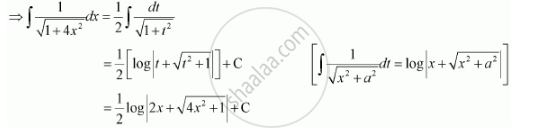Share

# Integrate the Functions 1/Sqrt(1+4x^2) - Mathematics

#### Question

Integrate the functions 1/sqrt(1+4x^2)

#### Solution

Let 2x = t

∴ 2dx = dtIs there an error in this question or solution?

#### Video TutorialsVIEW ALL 

Solution Integrate the Functions 1/Sqrt(1+4x^2) Concept: Integrals of Some Particular Functions.
S